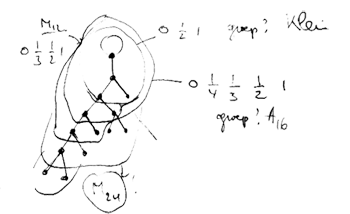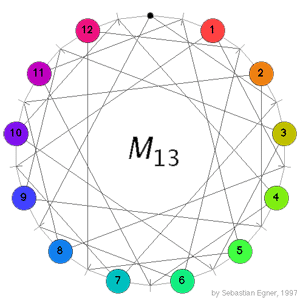# Tag: Brauer

Bruce Westbury has a page on recent work on series of Lie groups including exceptional groups. Moreover, he did put his slides of a recent talk (probably at MPI) online.

Probably, someone considered a similar problem for simple groups. Are there natural constructions leading to a series of finite simple groups including some sporadic groups as special members ? In particular, does the following sequence appear somewhere ?

$L_2(7), M_{12}, A_{16}, M_{24}, A_{28}, A_{40}, A_{48}, A_{60}, \ldots$

Here, $L_2(7)$ is the simple group of order 168 (the automorphism group of the Klein quartic), $M_{12}$ and $M_{24}$ are the sporadic Mathieu groups and the $A_n$ are the alternating simple groups.

I’ve stumbled upon this series playing around with Farey sequences and their associated ‘dessins d’enfants’ (I’ll come back to the details of the construction another time) and have dubbed this sequence the Iguanodon series because the shape of the doodle leading to its first few termsreminded me of the Iguanodons of Bernissart (btw. this sketch outlines the construction to the experts). Conjecturally, all groups appearing in this sequence are simple and probably all of them (except for the first few) will be alternating.

I did verify that none of the known low-dimensional permutation representations of other sporadic groups appear in the series. However, there are plenty of similar sequences one can construct from the Farey sequences, and it would be nice if one of them would contain the Conway group $Co_1$. (to be continued)Conway’s puzzle M(13) is a variation on the 15-puzzle played with the 13 points in the projective plane $\mathbb{P}^2(\mathbb{F}_3)$. The desired position is given on the left where all the counters are placed at at the points having that label (the point corresponding to the hole in the drawing has label 0). A typical move consists in choosing a line in the plane going through the point where the hole is, choose one of the three remaining points on this line and interchange the counter on it for the hole while at the same time interchanging the counters on the other two points. In the drawing on the left, lines correspond to the little-strokes on the circle and edges describe which points lie on which lines. For example, if we want to move counter 5 to the hole we notice that both of them lie on the line represented by the stroke just to the right of the hole and this line contains also the two points with counters 1 and 11, so we have to replace these two counters too in making a move. Today we will describe the groupoid corresponding to this slide-puzzle so if you want to read on, it is best to play a bit with Sebastian Egner’s M(13) Java Applet to see the puzzle in action (and to use it to verify the claims made below). Clicking on a counter performs the move taking the counter to the hole.

Here a collection of pdf-files of NeverEndingBooks-posts on groups, in reverse chronological order.

The categorical cafe has a guest post by Tom Leinster Linear Algebra Done Right on the book with the same title by Sheldon Axler. I haven’t read the book but glanced through his online paper Down with determinants!. Here is ‘his’ proof of the fact that any n by n matrix A has at least one eigenvector. Take a vector $v \in \mathbb{C}^n$, then as the collection of vectors ${ v,A.v,A^2.v,\ldots,A^n.v }$ must be linearly dependent, there are complex numbers $a_i \in \mathbb{C}$ such that $~(a_0 + a_1 A + a_2 A^2 + \ldots + a_n A^n).v = \vec{0} \in \mathbb{C}^n$ But then as $\mathbb{C}$ is algebraically closed the polynomial on the left factors into linear factors $a_0 + a_1 x + a_2 x^2 + \ldots + a_n x^n = c (x-r_1)(x-r_2) \ldots (x-r_n)$ and therefore as $c(A-r_1I_n)(A-r_2I_n) \ldots (A-r_nI_n).v = \vec{0}$ from which it follows that at least one of the linear transformations $A-r_j I_n$ has a non-trivial kernel, whence A has an eigenvector with eigenvalue $r_j$. Okay, fine, nice even, but does this simple minded observation warrant the extreme conclusion of his paper (on page 18) ?

As mathematicians, we often read a nice new proof of a known theorem, enjoy the different approach, but continue to derive our internal understanding from the method we originally learned. This paper aims to change drastically the way mathematicians think about and teach crucial aspects of linear algebra.

The simple proof of the existence of eigenvalues given in Theorem 2.1 should be the one imprinted in our minds, written on our blackboards, and published in our textbooks. Generalized eigenvectors should become a central tool for the understanding of linear operators. As we have seen, their use leads to natural definitions of multiplicity and the characteristic polynomial. Every mathematician and every linear algebra student should at least remember that the generalized eigenvectors of an operator always span the domain (Proposition 3.4)‚Äîthis crucial result leads to easy proofs of upper-triangular form (Theorem 6.2) and the Spectral Theorem (Theorems 7.5 and 8.3).

Determinants appear in many proofs not discussed here. If you scrutinize such proofs, you‚Äôll often discover better alternatives without determinants. Down with Determinants!

I welcome all new proofs of known results as they allow instructors to choose the one best suited to their students (and preferable giving more than one proof showing that there is no such thing as ‘the best way’ to prove a mathematical result). What worries me is Axler’s attitude shared by extremists and dogmatics world-wide : they are so blinded by their own right that they impoverish their own lifes (and if they had their way, also that of others) by not willing to consider other alternatives. A few other comments :

1. I would be far more impressed if he had given a short argument for the one line he skates over in his proof, that of $\mathbb{C}$ being algebraically closed. Does anyone give a proof of this fact anymore or is this one of the few facts we expect first year students to accept on faith?

1. I dont understand this aversity to the determinant (probably because of its nonlinear character) but at the same time not having any problems with successive powers of matrices. Surely he knows that the determinant is a fixed $~\mathbb{Q}~$-polynomial in the traces (which are linear!) of powers of the matrix.

2. The essense of linear algebra is that by choosing a basis cleverly one can express a linear operator in a extremely nice matrix form (a canonical form) so that all computations become much more easy. This crucial idea of considering different bases and their basechange seems to be missing from Axler’s approach. Moreover, I would have thought that everyone would know these days that ‘linear algebra done right’ is a well developed topic called ‘representation theory of quivers’ but I realize this might be viewed as a dogmatic statement. Fortunately someone else is giving the basic linear algebra courses here in Antwerp so students are spared my private obsessions (at least the first few years…). In [his post](http://golem.ph.utexas.edu/category/2007/05/ linear_algebra_done_right.html) Leistner askes “What are determinants good for?” I cannot resist mentioning a trivial observation I made last week when thinking once again about THE rationality problem and which may be well known to others. Recall from the previous post that rationality of the quotient variety of matrix-couples $~(A,B) \in M_n(\mathbb{C}) \oplus M_n(\mathbb{C}) / GL_n$ under _simultaneous conjugation_ is a very hard problem. On the other hand, the ‘near miss’ problem of the quotient variety of matrix-couples ${ (A,B)~|~det(A)=0~} / GL_n$ is completely trivial. It is rational for all n. Here is a one-line proof. Consider the quiver $\xymatrix{\vtx{} \ar@/^2ex/[rr] & & \vtx{} \ar@(ur,dr) \ar@/^2ex/[ll]}$ then the dimension vector (n-1,n) is a Schur root and the first fundamental theorem of $GL_n$ (see for example Hanspeter Krafts excellent book on invariant theory) asserts that the corresponding quotient variety is the one above. The result then follows from Aidan Schofield’s paper Birational classification of moduli spaces of representations of quivers. Btw. in this special case one does not have to use the full force of Aidan’s result. Zinovy Reichstein, who keeps me updated on events in Atlanta, emailed the following elegant short proof Here is an outline of a geometric proof. Let $X = {(A, B) : det(A) = 0} \subset M_n^2$ and $Y = \mathbb{P}^{n-1} \times M_n$. Applying the no-name lemma to the $PGL_n$-equivariant dominant rational map $~X \rightarrow Y$ given by $~(A, B) \rightarrow (Ker(A), B)$ (which makes X into a vector bundle over a dense open $PGL_n$-invariant subset of Y), we see that $X//PGL_n$ is rational over $Y//PGL_n$ On the other hand, $Y//PGLn = M_n//PGL_n$ is an affine space. Thus $X//PGL_n$ is rational. The moment I read this I knew how to do this quiver-wise and that it is just another Brauer-Severi type argument so completely inadequate to help settling the genuine matrix-problem. Update on the paper by Esther Beneish : Esther did submit the paper in february.